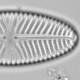## Testing Doug Keenan’s methods

Doug Keenan’s allegation that he has a confession from the Met Office that “statistically significant temperature rise can’t be supported” has been devoured with relish by the pigeons.

But elsewhere, Lucia has argued that

Doug is going on about the fact that a statistical model treating the of trendless data with ARIMA noise with d=1 appears to fit the data better linear trend+ ARIMA with d=0. It probably does so but that means very little because:
1) Physically no one expects the AGW forcings would have caused the trend to look like “straight line + noise” since 18whatever.
2) ARIMA with d=1 alone would violate the first law of thermo. (i.e. violates the 1st law of thermo. We don’t even need to get fancy and go to the 2nd.)

I want to briefly look at her first argument. I’m going to generate some simulated data that is linear trend + noise and test whether the linear trend model or Keenan’s ARIMA(3,1,0) has the lower Akaike information criterion (AIC). Lower AIC’s indicate a better model.

```x<-1:100
y<-rnorm(length(x), x, 10)#linear
plot(x,y)

AIC(lm(y~x))
AIC(arima(y, order=c(3,1,0)))
```

With the particular set of random data I used, the linear trend model had an AIC of 750, far below the ARIMA model’s AIC of 774. The linear trend model is the better model.

Now let’s try making the relationship slightly curved by adding a quadratic to the trend.

```x<-1:100
y<-rnorm(length(x), x+0.02*x^2, 10)#slight curve
plot(x,y)

AIC(lm(y~x))
AIC(arima(y, order=c(3,1,0)))
```

Now the linear model has an AIC of 877, far above the ARIMA model’s AIC of 809.

In this second test, the ARIMA(3,1,0) appears to be a far better model according to the AIC. Is the simulated data drawn from an ARIMA(3,1,0) process? No. Are the coefficients of the ARIMA model interpretable? No.

This demonstrates that Keenan’s test is very sensitive to deviations from the linear trend in the temperature record. That the global temperature has not had a linear trend over the instrumental period is not in the least unexpected as the climate forcing has not had a linear trend. It would seem a mistake to reject a linear trend model that nobody thinks is perfect in favour of an ARIMA(3,1,0) process that violates the first law of thermodynamics. Keenan should remember that AIC can only indicate the best model, from a purely statistical point of view, of those tested — there may be a much better but untested model.Ecologist with interests in quantitative methods and palaeoenvironments
This entry was posted in climate, Fake climate sceptics, R, Silliness, WUWT and tagged . Bookmark the permalink.

### 9 Responses to Testing Doug Keenan’s methods

1.lucia liljegren (@lucialiljegren) says:

Cool! Nick Stokes told me you wrote this. Before clicking over I said this sounded like the sort of test I like to do!

2.ucfagls says:

HI Richard, Are you sure `lm()` and `arima()` are comparable? The former doesn’t use ML at all, whereas the latter does. One usually has to be very careful that the same additive constant is used in the likelihood. Is that the case here?

•richard telford says:

Hei Gavin, I would certainly be cautious about the AIC being correct. I assume I am replicating Keenan’s methods, so if there is a problem here he has a problem too.

3.James says:

Your “slight curve” has a slope that increases by a factor of 5.

•richard telford says:

So a change in slope that is rather small compared with the changes in slope in the temperature record.

4.lucia liljegren (@lucialiljegren) says:

The aic from arima are consistent and give the same results as Keenan’s method to within an additive constant. I think Keenan used corrected AICc
calcAICc<- function(aicx,n,k) aicx+(2*k*(k+1))/(n-(k+1))

•ucfagls says:

I was meaning whether it was appropriate to compare a model fitted via `lm()` with one fitted via `arima()` using AIC if the two approaches use different constants in their likelihood.